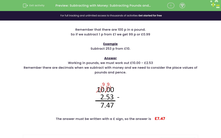# Subtracting with Money: Subtracting Pounds and Pence

In this worksheet, students subtract the given amounts of money, giving their answers in pounds.Key stage:  KS 2

Curriculum topic:   Measurement

Curriculum subtopic:   Solve Four Operations Problems

Popular topics:   Place Value worksheets

Difficulty level:#### Worksheet Overview

Remember that there are 100 p in a pound.

So if we subtract 1 p from £1 we get 99 p or £0.99

Example

Subtract 253 p from £10.

Working in pounds, we must work out £10.00 - £2.53

Remember there are decimals when we subtract with money and we need to consider the place values of pounds and pence.The answer must be written with a £ sign, so the answer is    £7.47

### What is EdPlace?

We're your National Curriculum aligned online education content provider helping each child succeed in English, maths and science from year 1 to GCSE. With an EdPlace account you’ll be able to track and measure progress, helping each child achieve their best. We build confidence and attainment by personalising each child’s learning at a level that suits them.

Get started